### Home > CALC > Chapter 6 > Lesson 6.1.1 > Problem6-13

6-13.
1. Evaluate each limit. Homework Help ✎

1.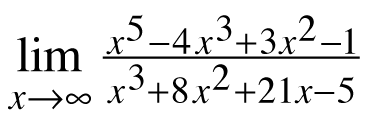2.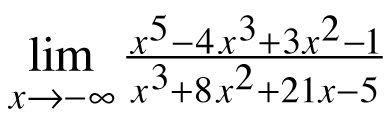3.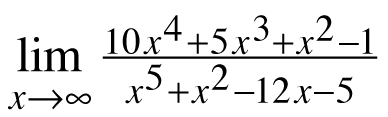4.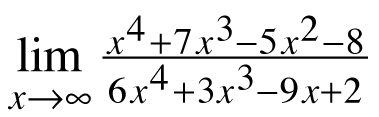5.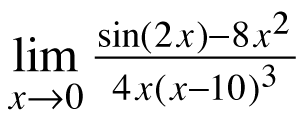6.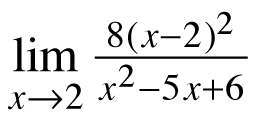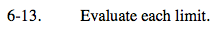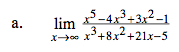To find the limit as the function approaches ∞, look at the end behavior of the function.

Find the end behavior by looking at only the highest-power terms in the denominator and numerator.

$=\lim_{x\rightarrow \infty }\frac{x^{5}}{x^{3}}=\lim_{x\rightarrow \infty }x^{2}=$

Because the function's end behavior follows the trend of an x² graph, we know that the limit as the function approaches ∞ is also ∞.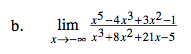This is ALMOST the same problem as part (a) but, evaluate the limit as x→−∞ instead of ∞.
Note: sometimes there is are different end-behavior limits in each direction.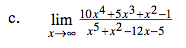Refer to part (a). Remember to include 10 as part of the highest power for the numerator!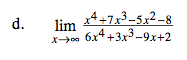See part (a).

$=(\text{show steps})=\frac{1}{6}.$

Note: this means that there is a horizontal asymptote of

$y=\frac{1}{6}.$